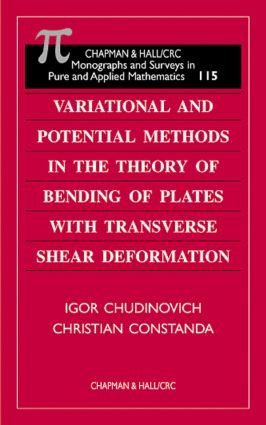Variational and Potential Methods in the Theory of Bending of Plates with Transverse Shear Deformation

1st Edition

Chapman and Hall/CRC

248 pages

Purchasing Options:\$ = USD
Hardback: 9781584881551
pub: 2000-06-13
SAVE ~\$37.00
\$185.00
\$148.00
x

FREE Standard Shipping!

Description

Elastic plates form a class of very important mechanical structures that appear in a wide range of practical applications, from building bodies to microchip production. As the sophistication of industrial designs has increased, so has the demand for greater accuracy in analysis. This in turn has led modelers away from Kirchoff's classical theory for thin plates and toward increasingly refined models that yield not only the deflection of the middle section, but also account for transverse shear deformation. The improved performance of these models is achieved, however, at the expense of a much more complicated system of governing equations and boundary conditions.

In this Monograph, the authors conduct a rigorous mathematical study of a number of boundary value problems for the system of partial differential equations that describe the equilibrium bending of an elastic plate with transverse shear deformation. Specifically, the authors explore the existence, uniqueness, and continuous dependence of the solution on the data. In each case, they give the variational formulation of the problems and discuss their solvability in Sobolev spaces. They then seek the solution in the form of plate potentials and reduce the problems to integral equations on the contour of the domain.

This treatment covers an extensive range of problems and presents the variational method and the boundary integral equation method applied side-by-side. Readers will find that this feature of the book, along with its clear exposition, will lead to a firm and useful understanding of both the model and the methods.

Reviews

"It is amazing that the authors have managed to cover so many fundamental boundary-value problems and present the variational method and the boundary integral equation method applied side-by-side in a single volume…This feature of the book will certainly strengthen understanding of both the model and the methods. The writing style is very clear, the book is self-contained and easy to read, and it should be extremely valuable to researchers interested in applied analysis and mathematical models in elasticity."

-Proceedings of the Edinburgh Mathematical Society (2002, vol. 45)

"This book will be useful for mathematicians, theoretical engineers, and all interested in mathematical modeling in elasticity."

-European Mathematical Society Newsletter, No. 40 (June 2001)

Introduction

FORMULATION OF THE PROBLEMS

The Equilibrium Equations for Plates

The Boundary Value Problems

The Plate Potentials and their Properties

Boundary Integral Equations

VARIATIONAL FORMULATION OF THE DIRICHLET AND NEUMANN PROBLEMS

Function Spaces

Solvability of the Interior Problems

Weighted Sobolev Spaces

Solvability of the Exterior Problems

BOUNDARY INTEGRAL EQUATIONS FOR THE DIRICHLET AND NEUMANN PROBLEMS

The Area Potential and its Properties

The Poincaré-Steklov Operators

Further Properties of the Plate Potentials

Solvability of the Boundary Equations

TRANSMISSION BOUNDARY VALUE PROBLEMS

Formulation and Solvability of the Problems

Infinite Plate with a Finite Inclusion

Multiply Connected Finite Plate

Finite Plate with an Inclusion

PLATE WEAKENED BY A CRACK

Formulation and Solvability of the Problems

The Poincaré-Steklov Operator

The Single Layer and Double Layer Potentials

Infinite Plate with a Crack

Finite Plate with a Crack

BOUNDARY VALUE PROBLEMS WITH OTHER TYPES OF BOUNDARY CONDITIONS

Mixed Boundary Conditions

Boundary Equations for Mixed Conditions

Combined Boundary Conditions

Elastic Boundary Conditions

PLATE ON A GENERALIZED ELASTIC FOUNDATION

Formulation and Solvability of the Problems

A Fundamental Matrix of Solutions

Properties of the Boundary Operators

Solvability of the Boundary Equations

APPENDIX: An Elementary Introduction to Sobolev Spaces